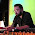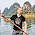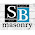# OpenCL

This site contains OpenCL notes, tutorials, benchmarks, news.

## Thursday, May 30, 2013

### Atomic operations and floating point numbers in OpenCL

Many times I had questions myself why atomic operations are not supported on floating point numbers. There are two reasons for that:
1. floating point approximation
2. hardware costs
What means the first reason? OpenCL doesn't define thread scheduling so this means that the order of the threads can be arbitrary. If we would use atomics that means that order of the arithmetic operations would be arbitrary too. In case of floating points it would cause the arbitrary results too what nobody wants. You don't believe? Let's take a look at the next example:

```float sum=0;
for(int i=0;i<10000000;i++){
sum+=1.0f;
}
sum+=100000000.0f;
std::cout<<std::setprecision(20) << "sum is: "<<sum<<"\n";
```
```float sum=0;
float sum=100000000.0f;
for(int i=0;i<10000000;i++){
sum+=1.0f;
}
std::cout<<std::setprecision(20) << "sum is: "<<sum<<"\n";
```

Now the question for 1M\$. What will the first cout print out and what second one? Looks like that booth should print 110000000 but this is not the case. Only the first one prints the expected result. The second cout prints 100000000. Why? Floating point numbers have 32 bits to store the numbers. To support big dynamic ranges of floating point number from −10308 through +10308 we need to store floating points as pair of mantissa and exponent. Number 100000000 from the second case can be internally stored as 1.0*10^8 (1.0 is mantissa, 10^8 is exponent). When we add small ones to very big value (1.0*10^8 + 1.0*10^0), the problem we get is how to represent the number 100000001 with only 32 bits? In our case the ones are simply ignored as small one is really not important against the big number 1.0*10^8.
In the first case we get correct result as on the line before print we simply sum 1.0*10^7 and 1.0*10^8. Then we get 11*10^7. As you might notice it seems that 9999998 + 1 can be represented with 32bit floating point number.

What about the second reason for atomic operations? The hardware costs. It's well known that integer arithmetic unit requires much less transistors than floating point arithmetic unit. Atomic arithmetic operations on the GPU can be implemented in two ways:
1. serialization of the memory operations
2. utilizing arithmetic unit in the memory controller or in the special queue
First one is simple to do but it is really slow as all threads which access the same memory location need to serialize. But at least atomic operations work.

Second way is the preferred one but it requires more transistors. To support fast atomic we need some kind of queue where we send the commands like "add value 5 to memory location XXXX". This way requires additional arithmetic units in special unit or at the memory controller.

As floating point arithmetic units are more costly there is no economical reason to include them into the special units which will be not utilized most of the time. You would probably use atomics only in rare cases, or?

Now you know why OpenCL has no atomic operations on floating point numbers. If you still like to have them you can serialize the memory access like it is done in the next code:

```float sum=0;

void atomic_add_global(volatile global float *source, const float operand) {
union {
unsigned int intVal;
float floatVal;
} newVal;
union {
unsigned int intVal;
float floatVal;
} prevVal;

do {
prevVal.floatVal = *source;
newVal.floatVal = prevVal.floatVal + operand;
} while (atomic_cmpxchg((volatile global unsigned int *)source, prevVal.intVal, newVal.intVal) != prevVal.intVal);
}
```

```float sum=0;
void atomic_add_local(volatile local float *source, const float operand) {
union {
unsigned int intVal;
float floatVal;
} newVal;

union {
unsigned int intVal;
float floatVal;
} prevVal;

do {
prevVal.floatVal = *source;
newVal.floatVal = prevVal.floatVal + operand;
} while (atomic_cmpxchg((volatile local unsigned int *)source, prevVal.intVal, newVal.intVal) != prevVal.intVal);
}
```

I found this code one the next blog: http://suhorukov.blogspot.com/2011/12/opencl-11-atomic-operations-on-floating.html . Many thanks.

First function works on global memory the second one work on the local memory. The only difference is the global/local word.

How this code works? It uses union which mean that we have value at memory location X which can be accessed as the integer or as floating point number. Union replaces type casting of pointers.

Next you see the do while loop which actually serializes the memory access. Function atomic_cmpxchg writes sum of value at memory location X and our operand to location X. At the same time it checks if any other thread wrote at the same location. If this is the case then we need to repeat the do while loop. You can see that this approach can get very slow especially if we write to same location from many threads.

If you would like to have atomic_mul or div you can simply replace + with your operator (/, *, -).

Be warned, this is slow as we figured out before!

1.Interestingly, there is an atomicAdd() for floats in CUDA. I have used it and it performs well. With opencl, I tried the code in the blog you mentioned the link and with it I get "Program build failure" when creating a program from a compiled bitcode (the atomic_cmpxchg() call is causing the problem, but I am not sure why (maybe the cast is the problem).
not sure why.

2.actually, I'm an idiot. It was another iffy function call that was causing the build failure. I've got the atomic add working and it is OK, but the cuda version of this code is faster.

3.atomics work great in cuda. The serialization is dependant on the contention at a given address.

4.Professionally written blogs are rare to find, however I appreciate all the points mentioned here. I also want to include some other writing skills which everyone must aware of.
รักษาโรคไมเกรน

5.c++ programming example codes
cplusplus | String operation

6.replica rolex Orologi, che combina uno stile elegante e una tecnologia all'avanguardia, una varietà di stili di replica rolex rolex deepsea Orologi, il puntatore cammina tra il tuo stile di gusto esclusivo.